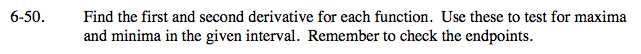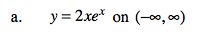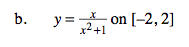### Home > CALC > Chapter 6 > Lesson 6.1.4 > Problem6-50

6-50.Since this is an OPEN interval, you are checking for LOCAL maxima and minima only.

You can use the 1st- or the 2nd-Derivative Test.
No matter which test you choose, your first step will be to find extrema CANDIDATES by determining where y' = 0 AND where y' = DNE.

1st-Derivative Test: Check values to the left and the right of each candidate.
If f '(x) changes from positive to negative, then you found a local maximum.
If f '(x) changes from negative to positive, then you found a local minimum.
If f '(x) does not change sign, then you found a point of inflection.

2nd-Derivative Test: Evaluate each candidate in the 2nd-derivative.
If f ''(candidate) is negative, then you found a local maximum.
If f ''(candidate) is positive, then you found a local minimum.
If f ''(candidate) = 0 or DNE, then this test is inconclusive.

There is a local minimum at x = −1.
But recall that a local min or max is a y-value, not an x-value.Since this is a CLOSED interval, you are checking for both LOCAL and GLOBAL maxima and minima.

Find the local maxima and minima. (Refer to the hints in part (a) for guidance.)
Note: These are also global candidates.

Find the global max and min by evaluating the y-value of each candidate.
The highest y-value is the global maximum.
The lowest y-value is the global minimum.

It is very important to consider candidates where
y' = DNE.
Recall that a derivative will not exist at a cusp, endpoint, jump, hole or vertical tangent.
SO CONSIDER THE ENDPOINTS AS CANDIDATES.